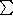## CGAL::Vector_d<Kernel>

### Definition

An instance of data type Vector_d<Kernel> is a vector of Euclidean space in dimension d. A vector r = (r0,...,r d - 1) can be represented in homogeneous coordinates (h0,...,hd) of number type RT, such that ri = hi/hd which is of type FT. We call the ri's the Cartesian coordinates of the vector. The homogenizing coordinate hd is positive.

This data type is meant for use in computational geometry. It realizes free vectors as opposed to position vectors (type Point_d). The main difference between position vectors and free vectors is their behavior under affine transformations, e.g., free vectors are invariant under translations.

### Types

 Vector_d::LA the linear algebra layer. Vector_d::Cartesian_const_iterator a read-only iterator for the Cartesian coordinates. Vector_d::Homogeneous_const_iterator a read-only iterator for the homogeneous coordinates. Vector_d::Base_vector construction tag.

### Creation

Vector_d<Kernel> v;
introduces a variable v of type Vector_d<Kernel>.

Vector_d<Kernel> v ( int d, Null_vector);
introduces the zero vector v of type Vector_d<Kernel> in d-dimensional space. For the creation flag CGAL::NULL_VECTOR can be used.

template <class InputIterator>
Vector_d<Kernel> v ( int d, InputIterator first, InputIterator last);
introduces a variable v of type Vector_d<Kernel> in dimension d. If size [first,last) == d this creates a vector with Cartesian coordinates set [first,last). If size [first,last) == p+1 the range specifies the homogeneous coordinates H = set [first,last) = ( ± h0, ± h1, ..., ± hd) where the sign chosen is the sign of hd.
 Precondition: d is nonnegative, [first,last) has d or d+1 elements where the last has to be non-zero.
 Requirement: The value type of InputIterator is RT.

template <class InputIterator>
Vector_d<Kernel> v ( int d, InputIterator first, InputIterator last, RT D);
introduces a variable v of type Vector_d<Kernel> in dimension d initialized to the vector with homogeneous coordinates as defined by H = set [first,last) and D: ( ± H, ± H, ..., ± H[d-1], ± D). The sign chosen is the sign of D.
 Precondition: D is non-zero, the iterator range defines a d-tuple of RT.
 Requirement: The value type of InputIterator is RT.

Vector_d<Kernel> v ( int d, Base_vector, int i);
returns a variable v of type Vector_d<Kernel> initialized to the i-th base vector of dimension d.
 Precondition: 0i < d.

Vector_d<Kernel> v ( RT x, RT y, RT w = 1);
introduces a variable v of type Vector_d<Kernel> in 2-dimensional space.
 Precondition: w0.

Vector_d<Kernel> v ( RT x, RT y, RT z, RT w);
introduces a variable v of type Vector_d<Kernel> in 3-dimensional space.
 Precondition: w0.

### Operations

int v.dimension () returns the dimension of v.

FT v.cartesian ( int i) returns the i-th Cartesian coordinate of v.
 Precondition: 0i < d.

FT v [ int i ] returns the i-th Cartesian coordinate of v.
 Precondition: 0i < d.

RT v.homogeneous ( int i) returns the i-th homogeneous coordinate of v.
 Precondition: 0id.

FT v.squared_length () returns the square of the length of v.

Cartesian_const_iterator v.cartesian_begin () returns an iterator pointing to the zeroth Cartesian coordinate of v.

Cartesian_const_iterator v.cartesian_end () returns an iterator pointing beyond the last Cartesian coordinate of v.

Homogeneous_const_iterator v.homogeneous_begin () returns an iterator pointing to the zeroth homogeneous coordinate of v.

Homogeneous_const_iterator v.homogeneous_end () returns an iterator pointing beyond the last homogeneous coordinate of v.

Direction_d<Kernel> v.direction () returns the direction of v.

Vector_d<Kernel> v.transform ( Aff_transformation_d<Kernel> t)
returns t(v).

### Arithmetic Operators, Tests and IO

 Vector_d& v *= RT n multiplies all Cartesian coordinates by n. Vector_d& v *= FT r multiplies all Cartesian coordinates by r. Vector_d v / RT n returns the vector with Cartesian coordinates vi/n, 0i < d. Vector_d v / FT r returns the vector with Cartesian coordinates vi/r, 0i < d. Vector_d& v /= RT n divides all Cartesian coordinates by n. Vector_d& v /= FT r divides all Cartesian coordinates by r. FT v * w inner product, i.e.,0i < d vi wi, where vi and wi are the Cartesian coordinates of v and w respectively. Vector_d v + w returns the vector with Cartesian coordinates vi+wi, 0i < d. Vector_d& v += w addition plus assignment. Vector_d v - w returns the vector with Cartesian coordinates vi-wi, 0i < d. Vector_d& v -= w subtraction plus assignment. Vector_d - v returns the vector in opposite direction. bool v.is_zero () returns true if v is the zero vector.

### Downward compatibility

We provide all operations of the lower dimensional interface x(), y(), z(), hx(), hy(), hz(), hw().

 Vector_d RT n * v returns the vector with Cartesian coordinates n vi. Vector_d FT r * v returns the vector with Cartesian coordinates r vi, 0i < d.

### Implementation

Vectors are implemented by arrays of variables of type RT. All operations like creation, initialization, tests, vector arithmetic, input and output on a vector v take time O(v.dimension()). coordinate access, dimension() and conversions take constant time. The space requirement of a vector is O(v.dimension()).